# 4.50 A compressor in a turbojet engine consur of shaft power to handle 100 kg/s of...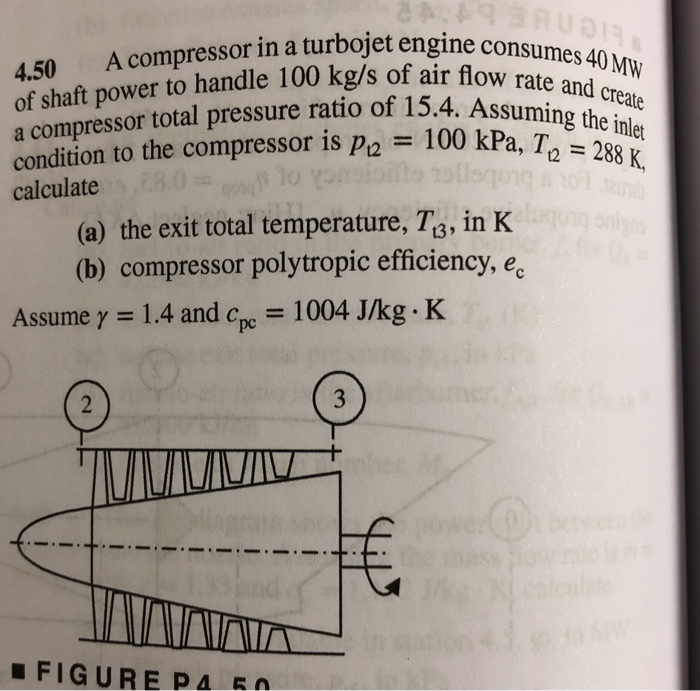4.50 A compressor in a turbojet engine consur of shaft power to handle 100 kg/s of air a compressor total pressure ratio of 15.4 rate and create e and cre 100 kPa, the nie , TO 288 K. condition to the compressor is p2 calculate (a) the exit total temperature, T3, in K (b) compressor polytropic efficiency, ec : 1.4 and cpc-1004 J/kg-K Assume γ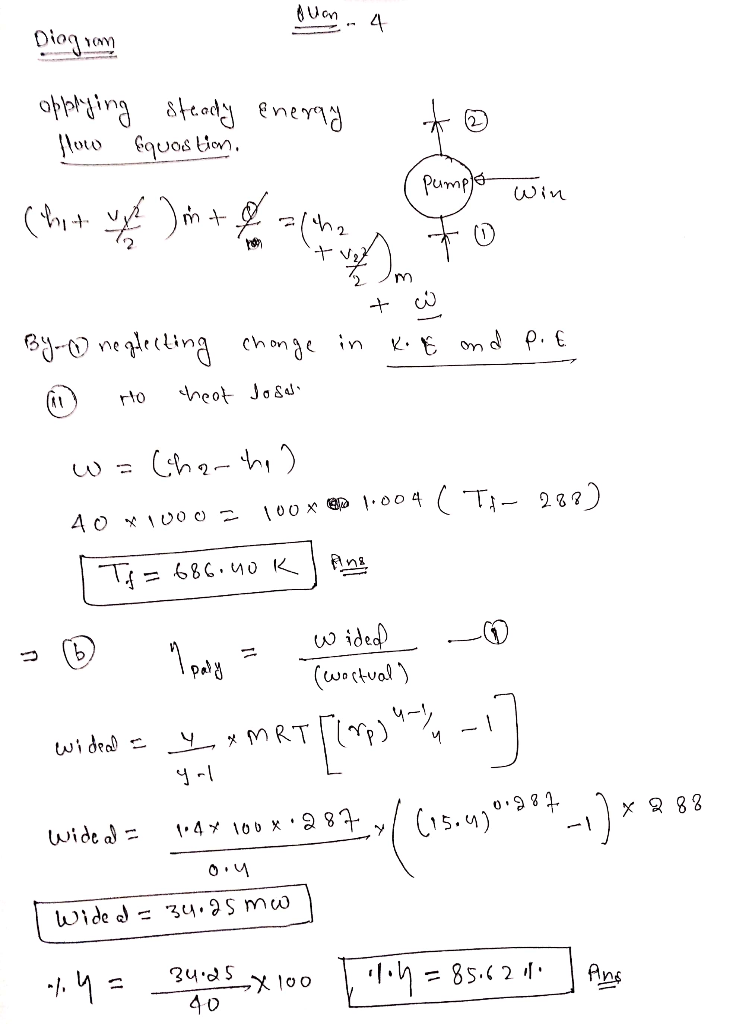##### Add Answer to: 4.50 A compressor in a turbojet engine consur of shaft power to handle 100 kg/s of...
Similar Homework Help Questions
• ### Problem-2 (200) Air at 30 kPa, 200 K, and 250 m/s enters a turbojet engine in...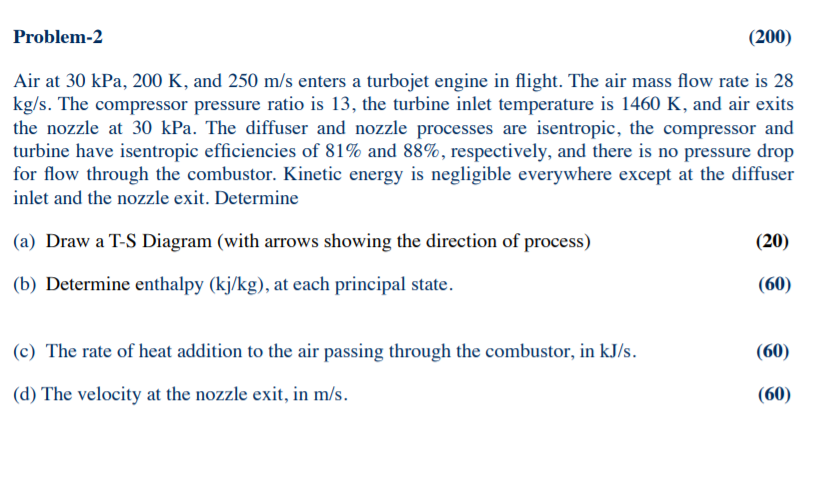Problem-2 (200) Air at 30 kPa, 200 K, and 250 m/s enters a turbojet engine in flight. The air mass flow rate is 28 kg/s. The compressor pressure ratio is 13, the turbine inlet temperature is 1460 K, and air exits the nozzle at 30 kPa. The diffuser and nozzle processes are isentropic, the compressor and turbine have isentropic efficiencies of 81% and 88%, respectively, and there is no pressure drop for flow through the combustor. Kinetic energy is negligible...

• ### A high-pressure axial compressor for a jet engine rotates at 15,000 rpm with an overall stagnation...

A high-pressure axial compressor for a jet engine rotates at 15,000 rpm with an overall stagnation pressure ratio of 8.5. The mass flow rate of air through the compressor is 16 kg/s and the stagnation conditions at inlet are 200 kPa and 450 K. The polytropic efficiency is 91%. a. If the mean radius is 0.24 m and this is constant throughout the compressor, calculate the total-to-total isentropic efficiency of the compressor and show that, for the stage loading to...

• ### 4.23 In a jet engine a flow of air at 1000 K, 200 kPa, and 30...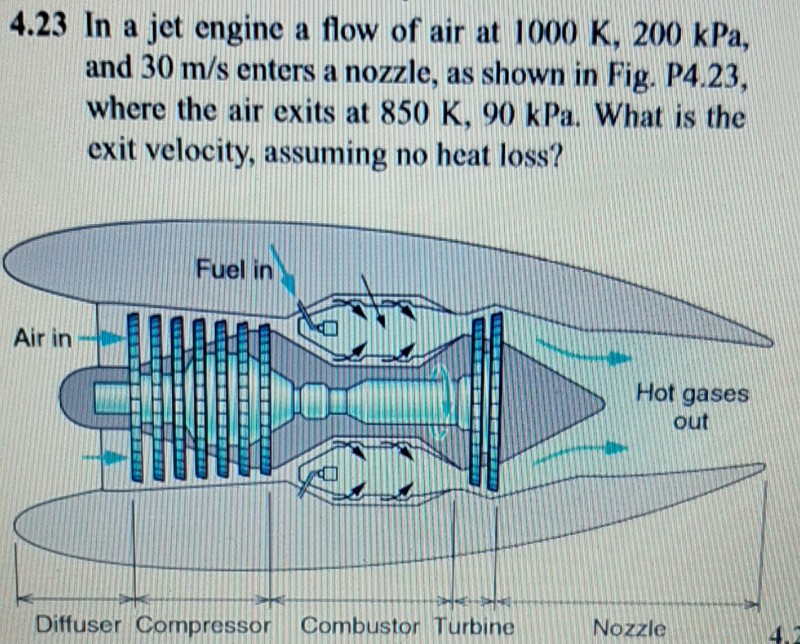4.23 In a jet engine a flow of air at 1000 K, 200 kPa, and 30 m/s enters a nozzle, as shown in Fig. P4.23, where the air exits at 850 K, 90 kPa. What is the exit velocity, assuming no heat loss? Fuel in Air in Hot gases out Diffuser Compressor Combuston Turbine Nozzle Carbon dioxide used as a natural refrigerant flows out of a cooler at 10 MPa. 40°C, after which it is throttled to 1.4 MPa. Find...

• ### QUESTION 2 A jet engine propels an aircraft at 289 m/s through air at 54 kPa and 267 x. The compressor pressure ratio is 9 and the temperature at the turbine inlet is 885 K. b) Taking the pressur...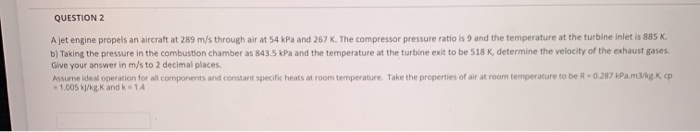QUESTION 2 A jet engine propels an aircraft at 289 m/s through air at 54 kPa and 267 x. The compressor pressure ratio is 9 and the temperature at the turbine inlet is 885 K. b) Taking the pressure in the combustion chamber as 843.5 kPa and the temperature at the turbine exit to be 518 K, determine the velocity of the exhaust gases. Give your answer in m/s to 2 decimal places Assume idesl operation for all components and...

• ### Use the following test results to estimate the overall isentropic efficiency of a single-sided ce...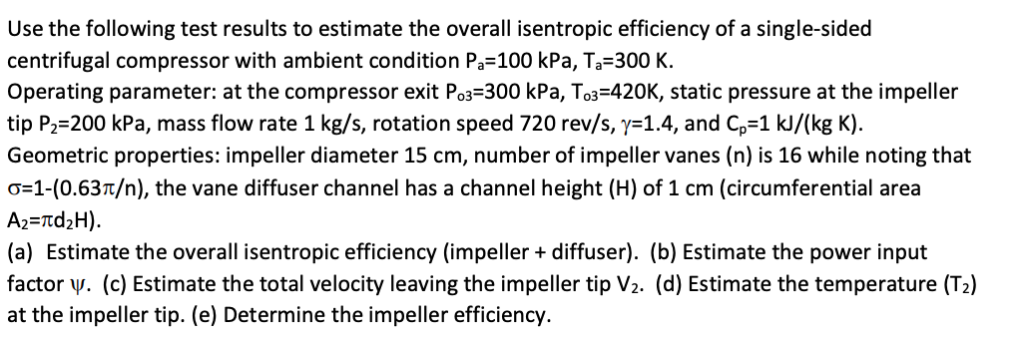Use the following test results to estimate the overall isentropic efficiency of a single-sided centrifugal compressor with ambient condition Pa-100 kPa, Ta-300 K. Operating parameter: at the compressor exit Po3-300 kPa, To3-420K, static pressure at the impeller tip P,-200 kPa, mass flow rate 1 kg/s, rotation speed 720 rev/s, γ=1.4, and C,-1 k /(kg K) Geometric properties: impeller diameter 15 cm, number of impeller vanes (n) is 16 while noting that G-1-(0.63T/n), the vane diffuser channel has a channel height...

Free Homework App